# Newton's third law – Examples

Example 1

For every action, there is an equal and opposite reaction.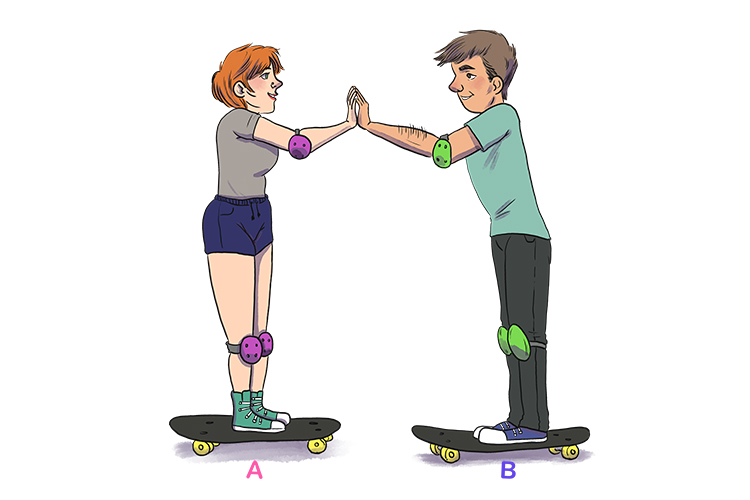Skater A pushes against skater B.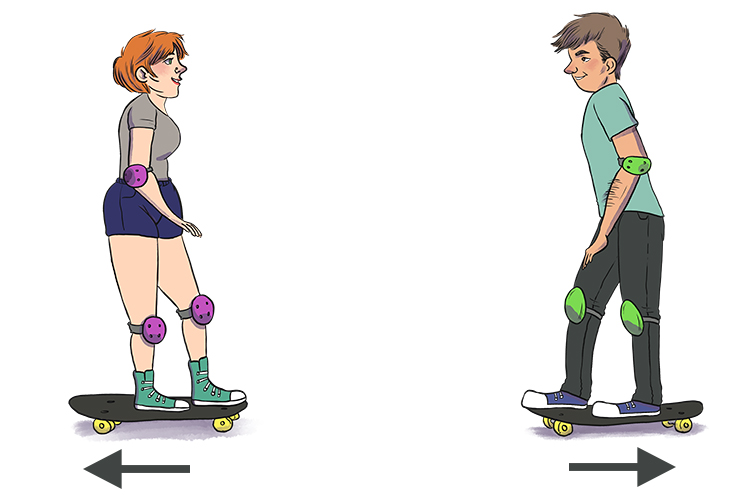Skater B will accelerate to the right according to F=ma

Skater A will accelerate to the left because there is an equal and opposite force.

Example 2

A woman on a boat tries to step off the boat on to a pier.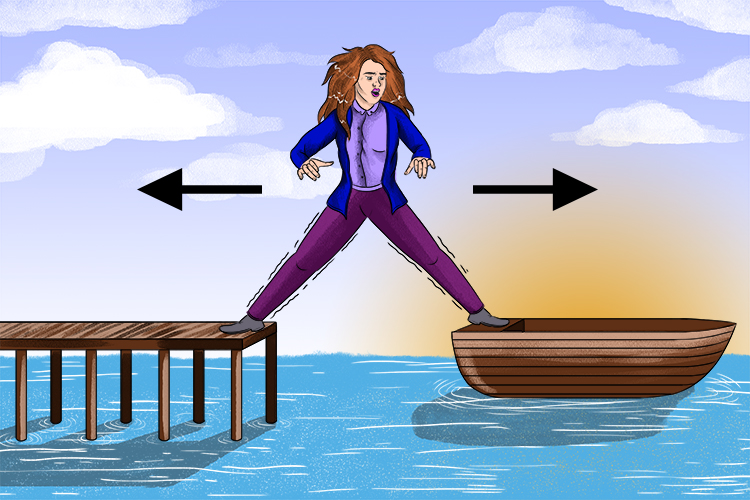For every action, there is an equal and opposite reaction. Pushing your body forward will have an equal reaction backwards on the boat.

NOTE: Forces always come in pairs: that is why Newton's third law is sometimes referred to as his law of pairs.

Example 3

NOTE: Before we go on with example 3 you need to know what a normal line is. It is an imaginary line perpendicular (90°) to a tangent line (in this case the surface). To remember this better see Mammoth Memory convex lenses and concave lenses.

Example 3 is a book being pushed across a table.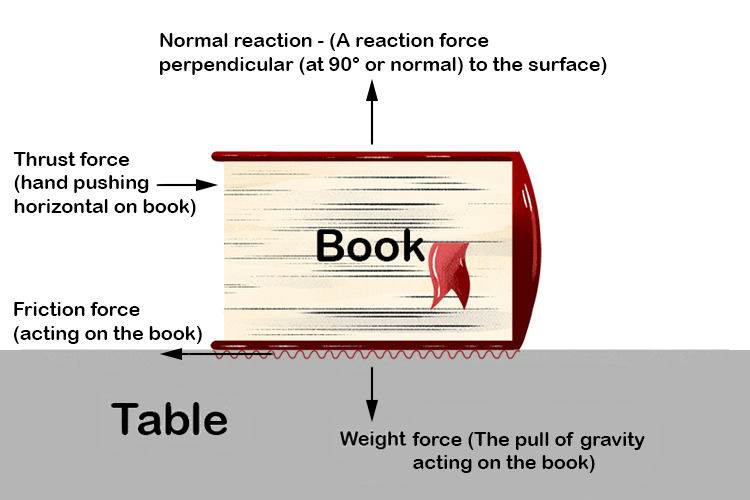This book being pushed along shows how forces come in pairs.

The book being pushed (thrust) has an opposing reaction of friction.

The weight of the book exerts a force downward and the table needs to exert an equal force upward or the table will collapse.

NOTE: You will note from the above that force is a vector, i.e. both magnitude and direction. The arrows denote the direction of the force.

NOTE: You will sometimes see the forces displayed as: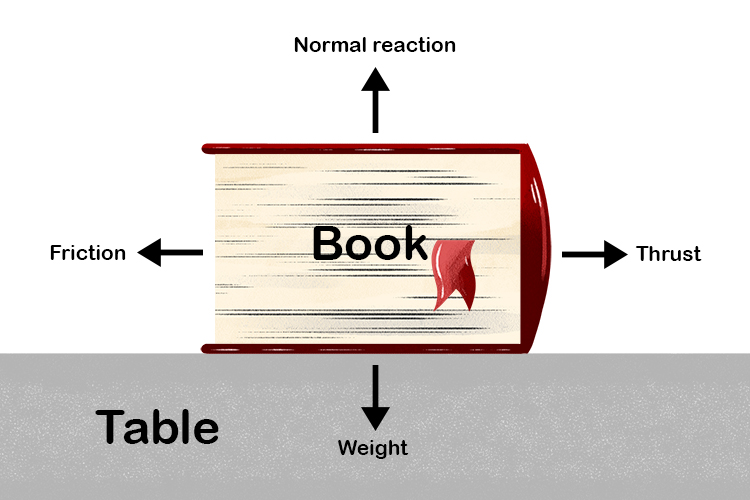Example 4

Releasing a balloon full of air has an equal and opposite reaction.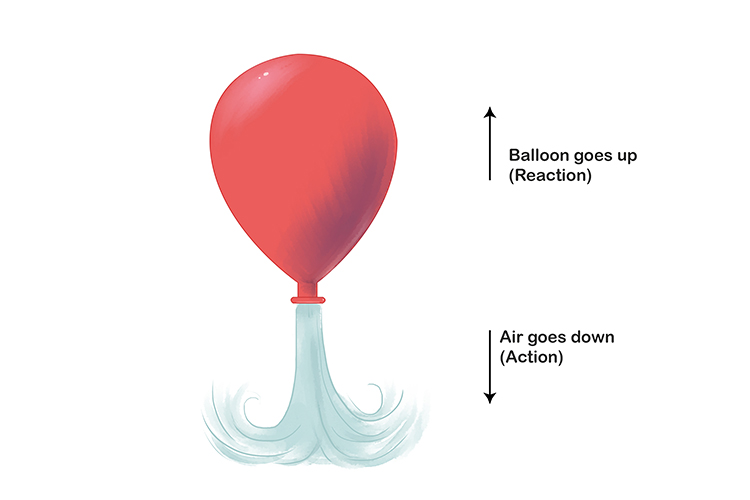Air is pushed out of the neck of the balloon but the balloon reacts in the opposite direction by moving upwards.

Example 5

A cannon firing.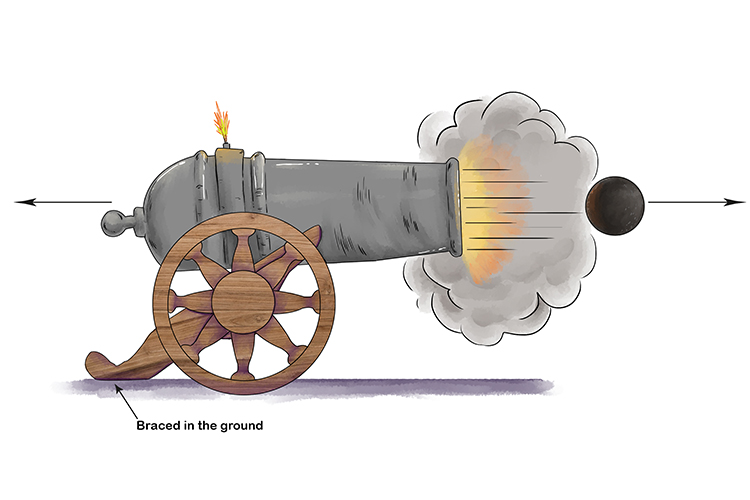The cannon exerting a force on a cannonball exhibits Newton's third law. Whenever an object exerts a force on a second object the second object exerts an equal and opposite force on the first object.

There will be an equal force on the cannon, but its larger mass and the bracing of the cannon in the ground, means it will not be kicked back too far.

Example 6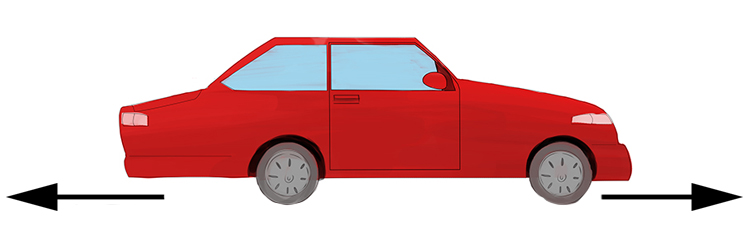A car travelling on a road. The tyres push forward on the road but the road pushes on the tyres. The two forces are equal and opposite.

Example 7

Firing a large gun on a skateboard.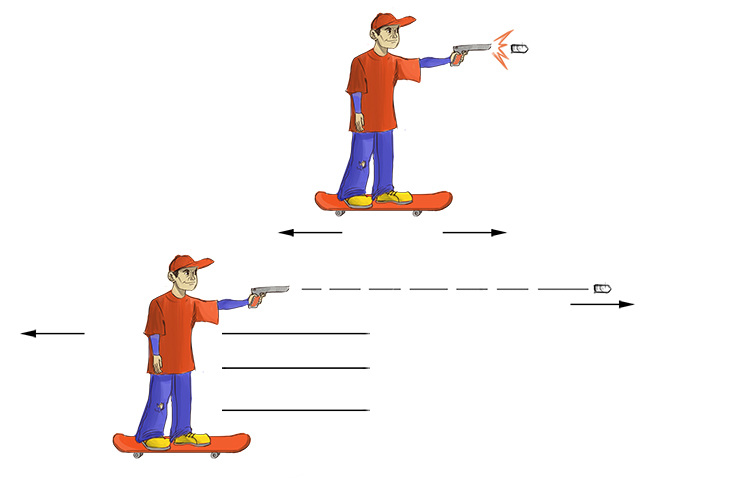If you fire a gun on a skateboard or even throw a medicine ball away from you on a skateboard, you will demonstrate Newton's third law. As you fire the bullet forward there is an equal and opposite reaction and the skateboard will move backwards.